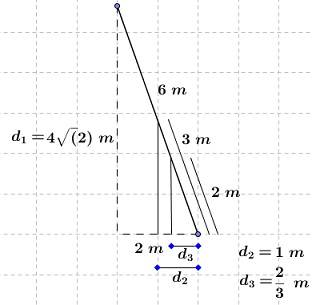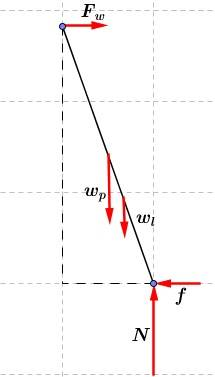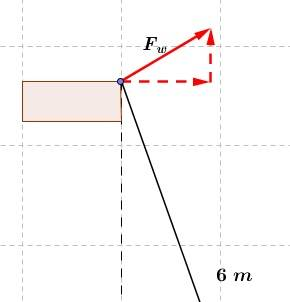## Homework StatementWhere it says ''from the bottom'' I assumed it's referring to a distance along the ladder. So:Data:

##w_{person} = 686\ N##

##d_1 = 4\sqrt(2)\ m##

##d_2 = 1\ m##

##d_3 = 2/3\ m##

## Homework Equations

##\sum \tau = 0##

##\sum F = 0##

## The Attempt at a Solution

[/B]
Diagram:I chose my pivot point to be the bottom of the ladder. Hence, torques due to normal force ##N## and friction ##f## are zero. ##ccw## is positive and ##cw## is negative.

##0 = w_pd_2 + w_ld_3 - F_wd_1##

##F_wd_1 = w_pd_2 + w_ld_3##

##F_w = \frac{w_pd_2 + w_ld_3}{d_1} = \frac{686\ N \cdot 1.00\ m + 98.0\ N \cdot 2/3\ m}{4\sqrt{2}\ m} = 133\ N##

##F_w = f = 133\ N##

Then:

##N = w_p + w_l = 686\ N + 98.0\ N = 784\ N##

The force at the top of the ladder is just ##133\ N##.
The force at the bottom is ##\small{\sqrt{N^2+ f^2} = \sqrt{ (784\ N)^2+ (133\ N)^2} = 795\ N}##. Doubt: this magnitude, whether it's correct or not, acts along the ladder, right? (as a tension would?).

The textbook's solutions are ##126\ N## and ##751\ N##, respectively. It seems I haven't missed anything, so I don't know where the mistake is.

Last edited:

BvU
Homework Helper
Which direction does Fw point ?

Which direction does Fw point ?

To the right.

And in a cw direction about the pivot point.

BvU
Homework Helper
No vertical component ?

No vertical component ?

Components of ##F_w##? Mmm, It didn't occur to me that there could be vertical components there. Has that of a ''frictionless rain gutter'' something to do with it?

It seems that I also forgot to take ##F_w## into account when finding the force at the bottom.

haruspex
Homework Helper
Gold Member
Components of ##F_w##? Mmm, It didn't occur to me that there could be vertical components there. Has that of a ''frictionless rain gutter'' something to do with it?
Frictionless says there will only be a normal force. Normal means perpendicular to the surface of contact. What direction will that be? You should assume the ladder projects slightly beyond the point of contact with the gutter.

BvU
Homework Helper
Components of ##F_w##? Mmm, It didn't occur to me that there could be vertical components there. Has that of a ''frictionless rain gutter'' something to do with it?
Consider a more extreme case where the ladder is at 45 degrees (or even less) wrt the ground. Would it lean ON the gutter, as well as against the gutter ?

I think I'm getting the idea. It would be something like this:However I'm not sure what is the correct direction that ##F_w## takes.

I think the sharp corner of the gutter can be viewed as a fulcrum. Should ##F_w## act perpendicular to the edge of the gutter, as bisecting it? If that were the case, my drawing wouldn't be totally accurate. Would it be in that direction, independent of the angle that the ladder makes with the ground?.

haruspex
Homework Helper
Gold Member
Should ##F_w## act perpendicular to the edge of the gutter, as bisecting it?
No. It might help to rotate the problem around to a more familiar set-up. Imagine a table on flat level ground, but the legs of the table are sharp wedges, meeting the ground at some odd angle. Which way would the normal force act?
More generally, as I wrote, normal means perpendicular to the contact plane. I'm not sure how contact planes are defined generally, but if either surface is differentiable then it will be the tangent plane which passes through the point of contact. Taking the ladder uprights to be rectangular in section, they have a differentiable surface. Or if you take them to be arbitrarily thin rods then it must be a plane containing that rod and containing the edge line of the gutter - there is only one such. Sounds complicated, but the underlying idea is fairly intuitive.

BvU
Homework Helper
Another approach: ladder pushes against gutter. In which direction ? Gutter pushes back, opposite direction (no friction).

If there were a rubber hose between the two, how would it compress ? To a vertical ellipse ? Or to an ellipse with the long axis parallel to the ladder ?

I would say to an ellipse with the long axis parallel to the ladder, which would mean that the force exerted by the gutter on the ladder is perpendicular to it.

In the other case (leaning against a wall), the wall becomes the differentiable surface and the rubber hose would compress parallel to it. It makes sense.

If the gutter weren't frictionless, friction would act parallel to the ladder's structure, right?

haruspex